Courses

# Adsorption kinetics Chemical Engineering Notes | EduRev

## Chemical Engineering : Adsorption kinetics Chemical Engineering Notes | EduRev

The document Adsorption kinetics Chemical Engineering Notes | EduRev is a part of Chemical Engineering category.
All you need of Chemical Engineering at this link: Chemical Engineering

Rate of adsorption : From kinetic theory the rate of collision, rc, is the rate at which molecules of a gas strike a bare surface per cm2 per sec at pressure p. The rc is given
as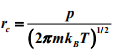molecules /cm.sec, where ‘kB’ is Boltzmanns constant, ‘m’ is mass and ‘T’ is temperature. Then the rate of adsorption is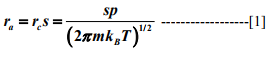The ‘s’ is known as the sticking coefficient and defined as fraction of molecules bombarding or impinging on the catalyst surface that stick to the surface on impact. The ‘s’ is also called the sticking probability. Now whether a molecule will be adsorbed (chemisorbed) depends on two main parameters: activation energy and configuration. Only those molecules possessing the required activation energy can be chemisorbed. Even if the molecules possess the necessary energy they may not be chemisorbed if the configuration of the molecule and surface site do not allow the activated complex to be traversed. The fraction of molecules possessing the required energy is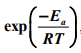,where Ea is the activation energy for chemisorption.

The configuration probability that a molecule will occupy a single site is proportional to the fraction of unoccupied surface, 1-θ, where θ is the fraction of surface covered. Therefore,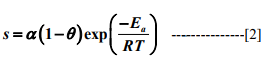α is the proportionality constant often known as ‘condensation coefficient’. It is the fraction of those molecules with energy greater than Ethat is adsorbed.

Combining equation  and  gives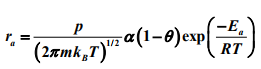or,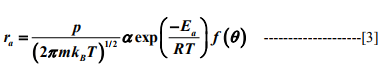For non- dissociative adsorption, f(θ)  fraction of surface uncovered = (1 - θ) For dissociative adsorption into two fragments,f (θ) = (1 - θ)2  and f(θ ) = (1-θ)3 −1 for three fragments. The type of adsorption depends on extent on interaction between adsorbate and adsorbent surface molecules.

In actual adsorption, the observed rate decreases rapidly with increasing surface coverage which implies that the activation energy increases with the surface coverage, θ. The condensation coefficient α also varies with θ. These variations are caused by surface heterogeneity, that is the activity of the adsorbent sites varies and different sites posses different values of α and Ea . The most active sites are associated with lowest activation energy. Hence probability of occupation of these sites by adsorbate molecules is higher. Further, the interaction between occupied and unoccupied site also affects the rate of adsorption. Hence α and Ea should be represented as function of θ.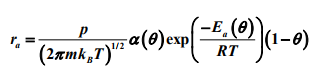Rates of desorption The rate of desorption is given by Polanyi –Wigner equation as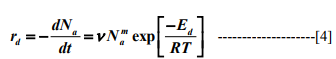where, Eis activation energy for desorption, ν is the pre-exponential factor of the desorption rate coefficient, Nis the number of binding sites for the adsorbed molecules and m is kinetic order for desorption process. Kinetic order suggests the nature of elementary steps that governs the desorption. A zero-order kinetics indicates that desorption occurs from multilayer where desorption is independent of coverage. A first order kinetics indicates the presence of single surface species whereas second order kinetics indicates recombination of adsorbate atoms leading to production of a diatomic molecule that is then desorbed.

Adsorption Isotherms and Isobars

When an adsorbate molecule in the gas phase comes in contact with the surface of the adsorbent, an equilibrium distribution of the adsorbate molecules takes place between the adsorbent surface and the gas phase. This equilibrium distribution depends upon various factors including partial pressure of adsorbate, temperature, nature of adsorbate, and the surface area and nature of adsorbent. Adsorption isotherm shows the amount of molecules adsorbed on the solid surface as a function of the equilibrium partial pressure at constant temperature.
Adsorption isobar shows the variation of adsorbed species with temperature at constant pressure.
The adsorption-desorption isotherm characterize the adsorbent. It depicts the nature of adsorption - desorption process occurring on the surface and also reveals the pore structure of the adsorbent. Often the isotherm is expressed as standard volume adsorbed as a function of relative pressure. Relative pressure is defined as the ratio of actual gas partial pressure over the saturated vapor pressure of adsorbate (P0) under constant temperature. By Brunauer empirical classification, five types of isotherms are observed for solid adsorbents as shown in Fig 1.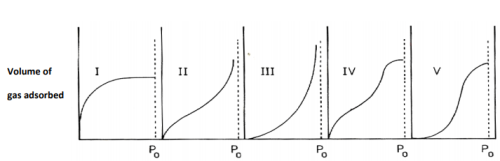Fig. 1. Types of adsorption isotherm according to Brunauer classification

Type I isotherm is for very small pores or microporous adsorbents. Adsorption occurs by filling of the micropores. The adsorbate uptake rate depends on the accessible micropore volume rather than total internal surface area. Type II and Type IV isotherms are observed for non-porous or macroporous adsorbents with unrestricted monolayer-multilayer adsorption. At first the adsorption volume rapidly increases at low relative pressures of less than 0.01 due to interaction of the adsorbate molecules with the higher energetic region followed by the interaction with less energetic region. When the monolayer formation of the adsorbed molecules is complete, multilayer formation starts to take place corresponding to the ‘sharp knee’ of the isotherms. As the relative pressure approaches unity an abrupt rise indicates the bulk condensation of adsorbate gas to liquid. Type III and Type V isotherms do not have the ‘sharp knee’ shape implying stronger adsorbate – adsorbate interactions than adsorbate-adsorbent interaction.

Hysteresis Loop
In case of isotherms for nonporous material, the desorption curve traces the adsorption curve. However, for the mesoporous and macroporous materials, desorption curve do not retrace the adsorption curve resulting in a wide loop. This is known as hysteresis loop and corresponds to the capillary condensation of adsorbate in the multilayer region, pore filling and emptying mechanism. The nature of the hysteresis loop is associated with different pore shapes and is shown in Fig 2.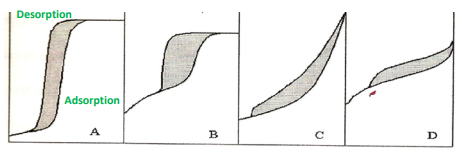Fig. 2. Different hysteresis loops as represented by IUPAC

Type A hysteresis is attributed to cylindrical or tubular type pores of adsorbent with a narrow distribution of uniform pores. It is characterized by steep and narrow parallel adsorption and desorption curves. Type B has a long flat plateau adsorption with a steep desorption curve. This is a complex structure of pores with interconnected networks or ink bottle shaped pores. Type C represents aggregates of adsorbent that contain parallel plates, slit- shape pores or wide capillaries (> 500 A). Type D is associated with slit- shape pores that are mainly in the micropore region.
Langmuir treatment of adsorption
Langmuir isotherm is derived based on the following assumptions:
1. Surface is energetically uniform, that is all the surface sites have the same activity for adsorption.
2. Adsorbed molecules do not interact with the other adsorbed molecules on the surface.
3. Heat of adsorption is therefore constant throughout the fractional surface coverage of 0 to 1.
4. Adsorption of all molecules occurs by the same mechanism and results in the same adsorbed structure.
5. Extent of adsorption is less than one complete monolayer coverage.
When a gas is in contact with a solid surface, the gas molecules continuously strike the surface and a fraction of these adhere. However, the more energetic molecules also continuously leave the surface. Eventually, the equilibrium is established so that the rate of adsorption equals the rate at which the molecules leave the surface.

Now, the rate of adsorption is equal to the rate of collision of molecules with the surface multiplied by a factor ‘s’ representing the fraction of the colliding molecules that adhere to the surface. At a given temperature, the rate of collision will be proportional to the partial pressure ‘p’ of the gas (its concentration) and the fraction ‘s’ will be constant. Hence, the rate of adsorption per unit of bare surface will be ra = rs = k΄p s = k p , where k is the constant involving ‘s’ and proportionality constant ‘k΄’.

Now, since the adsorption is limited to a monolayer coverage, the surface can be divided in two parts :
1. fraction θ covered by adsorbed molecule
2. fraction (1-θ ) which is bare Since only those molecules that strike the uncovered part of the surface can adsorb, the rate of adsorption per unit of total surface will also be proportional to (1-θ ). Therefore, the rate of adsorption is  rk p = (1 −θ) . Rate of desorption on the other hand is proportional to fraction of covered surface that is ' rk ''= θ

At equilibrium ,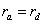or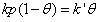or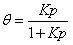where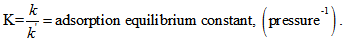.

Further θ can be written as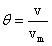where v = volume of gas adsorbed and vm = volume of gas adsorbed at monolayer coverage.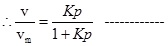This is known as the Langmuir isotherm. Among the empirical isotherms, only Type I isotherm ( Fig.1) agrees with the Langmuir equation. The Langmuir equation is valid for less than monolayer coverage and therefore more suited for chemisorption as chemisorption is limited to monolayer coverage. Deviations for other isotherms are mainly due to certain assumptions such as

2. no interaction between adsorbed molecules

1. 1. sites are of equal activity

These assumptions correspond to constant heat of adsorption. However for real systems, the heat of adsorption changes with surface coverage. The adsorption of H2 on metal films of Fe, Ni, W, drops with the increase in surface coverage. Two other well known isotherms, namely Temkin isotherms and Freundlich isotherm, may be derived in terms of dependency of heat of adsorption on fraction of surface coverage. The Temkin isotherms may be derived from the Langmuir isotherm by assuming that the heat of adsorption decreases linearly with increasing surface coverage θ. The result is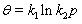where k1 and k2 are constant at given temperature. The Freundlich isotherm can be derived assuming a logarithmic decrease in heat of adsorption with surface coverage, i.e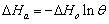. The isotherm is in the form of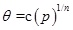. The value of ‘n' is generally greater than unity. The adsorption of H2 gas on tungsten follows this isotherm.

Derivation of adsorption isotherm from kinetics

An equation for adsorption isotherm can also be derived by equating the rates of adsorption and rate of desorption at equilibrium condition. From equations  and  at equilibrium,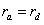. For the simplest case of non dissociative adsorption where f( θ ) = (1- θ ) and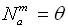. we obtain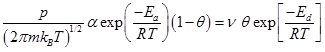Noting that Ed - Ea = - Δ H = heat of adsorption,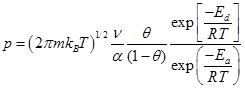or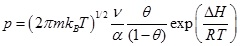Or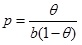where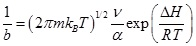If heat of adsorption remains constant with coverage, b is a function only of temperature and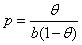or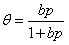-------------.

This equation  is the well known Langmuir isotherm.

Extended Langmuir model for adsorption in a multi-adsorbate system

The Langmuir model is also used to evaluate adsorption equilibrium data in a multi-adsorbate system and is known as extended Langmuir model. The extended Langmuir model mostly has been used to evaluate adsorption equilibrium data in binary system. The extended Langmuir model was first proposed by Butler and Ockrent . Their model was based on the same assumptions as that of Langmuir model for single adsorbates. That is the model assumed (i) a homogeneous surface with respect to the energy of adsorption, (ii) no interaction between adsorbed species, and (iii) that all adsorption sites are equally available to all adsorbed species. Assuming the rate of adsorption of a species at equilibrium is equal to its rate of desorption, the following equations were derived.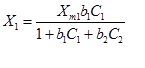--------------------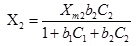--------------------

Where, the X1 and X2 are the amount of solute 1 and solute 2 respectively, which are absorbed per unit weight or per unit surface area of absorbent at equilibrium concentrations C1 and C2, respectively. The Xm1 and Xm2 are the maximum values of X1 and X2, respectively, that can be obtained in single solute system and correspond to monolayer coverage of the adsorbent. The b1and b2 are constants and function of the energy of adsorption of solutes 1 and 2, respectively, in a single solute system.

When some of the adsorption sites are inaccessible or unavailable to one type of solute in a bisolute system then, a fraction of the adsorption occurs without competition that is Xm1 ≠ Xm2. Jain and Snoeyink  proposed the following equations taking into account adsorptions with and without competition.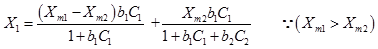------------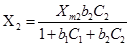------------------------------------------------------------

The first term on the right hand side of Eq. 9 is the number of molecules of solute 1 that adsorb without competition on the surface area proportional to (Xm1 -Xm2). The second term represents the number of solute 1 adsorbed on the surface area proportional to Xm2 under competition with solute 2 and is based on the Langmuir model for competitive adsorption. The number of molecules of solute 2 adsorbed on surface area proportional to Xm2 under competition with solute 1 can be calculated from Eq.10.

Offer running on EduRev: Apply code STAYHOME200 to get INR 200 off on our premium plan EduRev Infinity!

,

,

,

,

,

,

,

,

,

,

,

,

,

,

,

,

,

,

,

,

,

;